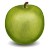# Counter

How many objects are in the figure below?A. $4$
B. $5$
C. $3$
D. $2$
E. $6$